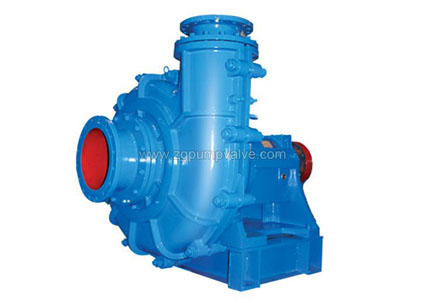# Guide To Selecting The Right Slurry Pump For Your Application

Learn - Jan. 05, 2022

Selecting a Slurry Pump:

1. Determine the flow rate

To size and select a pump, we first determine the flow rate.  In an industrial setting, the flow rate will often depend on the production level of the plant. Selecting the right flow rate may be as simple as determining that it takes 100 GPM (6.3 L/s) to fill a tank in a reasonable amount of time or the flow rate may depend on some interaction between processes that need to be carefully analyzed.

This is a matter of taking measurements of the height between the suction tank fluid surface and the discharge pipe end height or the discharge tank fluid surface elevation.

The friction head depends on the flow rate, the pipe size, and the pipe length. This is calculated from the values in the tables presented here. For fluids different than water the viscosity will be an important factor.

The total head is the sum of the static head (remember that the static head can be positive or negative) and the friction head.Double-Layer Slurry Pump

5. Select the pump

Pump selection is based using the total head and flow required as well as suitability to the application.

Slurry Pump Calculations

The following parameters must be determined when calculating a slurry pump.

Particle size and distribution

Particle size d50 (d85) is a measure of the percentage of particles in the slurry with a certain size or smaller.

The value is determined by sifting the solids through screens with varying mesh and then weighing each fraction. A sieve curve can then be drawn and the percentage of particles of different sizes read.

Ex: d85= 3 mm means that 85% of the particles have a diameter of 3 mm or less.

Mass fraction of small particles

The fraction of particles smaller than 75 µm. It is important to determine the percentage of small particles in the slurry. Particles smaller than 75 µm can to some extent facilitate the transport of larger particles. However, if the percentage of particles smaller than 75 µm exceeds 50%, the character of the slurry changes toward non-settling.

Solids

The density of the solids is stated as the Specific Gravity. This value, SGs, is determined by dividing the density of the solid by the density of water

Water

The density of water is 1000 kg/m3. The SG of water is 1,0 at 20°C. The value varies somewhat with

temperature.

Slurry

The specific gravity of the slurry can be determined using a nomograph.  Two of the values of SGs, Cv, and Cm, must be known

Particle shape

The shape of the particles is very significant for the behavior of the slurry when pumping and for the wear on the pump and the pipe system. The form factor denotes the deviation of the slurry particles from a perfect sphere.

Whenever pumps are used, it is important that the pump’s inlet pressure exceeds the vapor pressure of the liquid inside the pump. The necessary inlet pressure that is stated for the pump, NPSH req must not be less than the available value in the pump system, NPSH.

The available value depends on the ambient air pressure (height above sea level), the vapor pressure of the liquid, the density of the slurry, and the level in the sump.